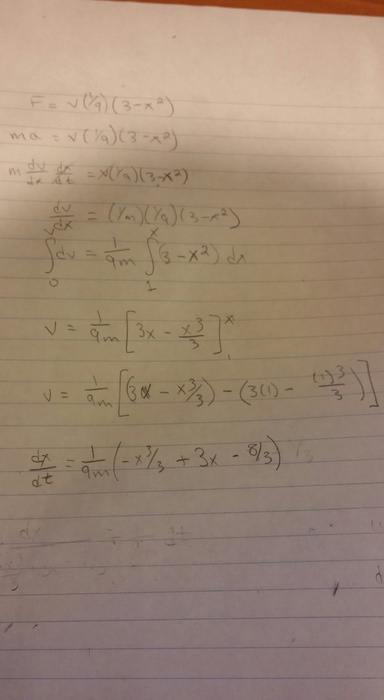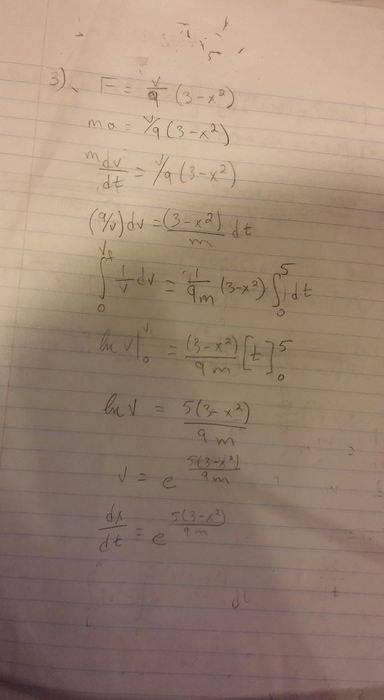# Classical Mechanics - finding displacement with given force

## Homework Statement

- The force acting on a particle m = 3kg is given by the following force equation: F = (v/9)(3 - x2),
the particle begins at a position of x = 1m with a speed of v = 0 m/s at time t = 0s. Find the displacement of the particle at time t = 5 s.

## Homework Equations

F = m(dv/dt) or F= m(dv/dx)(v)

## The Attempt at a Solution

Our professor set up the problem wrong, so he said all we have to do is get to the integral where we'd find x and stop.

Simon Bridge
Homework Helper
Welcome to PF;
... so what did you do?

Welcome to PF;
... so what did you do?
Well I tried it two ways, neither of which I thought was right...Simon Bridge
Homework Helper
In the first page, you cannot take the x's outside the integral over t because x is a function of time.
On the second page, you, instead, tried changing the variable ... that seems reasonable to me.
You'll end up with an implicit equation for x(t) but you only need x at a particular time.

In the first page, you cannot take the x's outside the integral over t because x is a function of time.
On the second page, you, instead, tried changing the variable ... that seems reasonable to me.
You'll end up with an implicit equation for x(t) but you only need x at a particular time.
So on the 2nd page you would end up with -(1/3)x3t + 3xt - (8/3)t ?

Simon Bridge